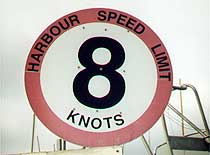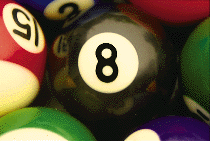# 8

= 2 × 2 × 2
A cube.

= 2 × 4

1, 1, 2, 3, 5, 8, 13...
A Fibonacci number.

Eight pints make a gallon.

Eight is the third number that stays the same when written upside down.

There are eight legs on a spider, barring accidents. Scorpions also have eight legs.

An eight is a racing boat with eight oars. Its crew is also called an eight. There are eight people in a tug-of-war team.

Some large car engines have eight cylinders.

According to Indian mythology, the Earth is supported on the backs of eight white elephants.

Before the rise of Christianity, there were eight days in the Greek and Roman weeks.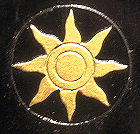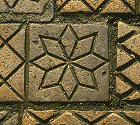Eight-fold symmetry is common in decoration.

Pieces of eight go with pirates and parrots. Originally they were piastre - Spanish silver coins of the seventeenth and eighteenth centuries marked with an '8' because they were worth eight reals. The dollar sign \$ is probably derived from the figure '8' as it appeared on 'pieces of eight'.

In the game of pool the Eight Ball is a black ball with the number 8. The expression 'behind the eight ball' means to be in a difficult or baffling situation.

A cube has eight corners or vertices.

Many words beginning oct- are related to the number eight. An octopus has eight arms and an octet is a group of eight musicians. (An octet of octopuses would therefore have 64 arms between them.)

An octagon is a figure with eight sides and an octahedron has eight faces.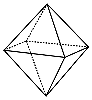Eight triangular faces on an octahedron.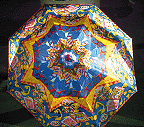Octagonal umbrellas are common but octagonal windows are not.

1 × 8 + 1 = 9
12 × 8 + 2 = 98
123 × 8 + 3 = 987
1 234 × 8 + 4 = 9 876
12 345 × 8 + 5 = 98 765
123 456 × 8 + 6 = 987 654
1 234 567 × 8 + 7 = 9 876 543
12 345 678 × 8 + 8 = 98 765 432
123 456 789 × 8 + 9 = 987 654 321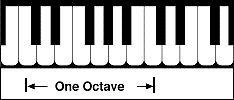An octave is the interval in music between two notes where the higher note has twice the frequency of the lower. On a piano keyboard this corresponds to an interval of eight white notes, for example, the notes C D E F G A B C.

Why is this eight notes rather than seven? We do not say a week has eight days because it starts and ends on a Sunday. Perhaps, to be logical, the interval should be called a septave rather than an octave? But when you come to play a musical scale it sounds incomplete unless you play all eight notes.

The term octave belongs to Western music, but the musical interval occurs in music around the world.

The amount of cloud in the sky is measured in oktas on a scale from zero to eight. 8 oktas means the sky is totally clouded and totally clear sky measures 0 oktas. A reading of 6 oktas would mean that three-quarters of the sky is overcast.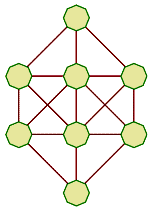Can you place the numbers from 1 to 8 in the cells of this diagram, so that no adjacent numbers are joined by a line?

For example, a line can join the numbers 5 and 8, because they are not adjacent, but the numbers 3 and 4 must not be connected by a line.